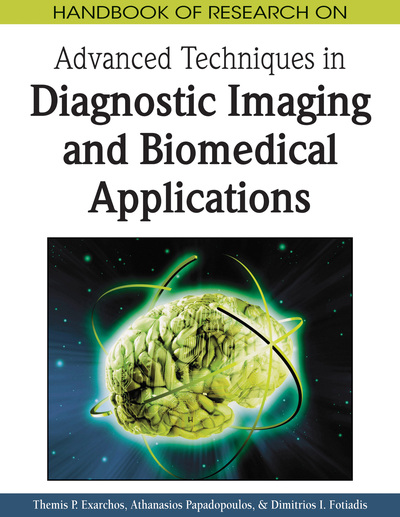# Segmentation of Cardiac Magnetic Resonance Images

Dimitrios G. Tsalikakis (University of Ioannina, Greece), Petros S. Karvelis (University of Ioannina, Greece) and Dimitrios I. Fotiadis (University of Ioannina, Greece)
DOI: 10.4018/978-1-60566-314-2.ch008
Available
\$37.50
No Current Special Offers

## Abstract

Segmentation plays a crucial role in cardiac magnetic resonance imaging (CMRI) applications, since it permits automated detection of regions of interest. In this chapter we review semi-automated and fully automated cardiac MRI segmentation techniques and discuss their advantages. We classify those segmentation methods as classical and model-based.
Chapter Preview
Top

## Background

CMRI can be acquired from several views. Long-axis (LA) and short-axis (SA) views of the heart are obtained routinely since random imaging planes can be selected. Figure 1 demonstrates the most common selected MRI views in a typical cardiovascular MR examination. CMRI mages are used to assess global and regional, RV and LV function as represented by systolic volume (SV), ejection fraction (EF), LV mass (Lorenz et al. 1999), wall-thickening (Vallejo et al. 2000), myocardial motion (Buckberg et al. 2007), and circumferential shortening of myocardial fibers (Tseng et al. 2003).

Figure 1.

Horizontal LA multi-segmented cine MR images during: (a) the end–diastolic phase and (b) the end–systolic phase. Vertical LA multi–segmented cine MR images during: (c) the end–diastolic phase and (d) the end–systolic phase. SA multi–segmented cine MR images during: (e) the end–diastolic phase and (f) the end–systolic phase.

Segmentation is the process of partitioning an image into regions which are homogeneous, related to one or more characteristics or features (Castleman, 1996). For the estimation of several cardiac functions during a CMRI examination, the boundaries of the LV, RV and epicardium need to be accurately determined. Thus, edge detection and image segmentation must be performed in order to generate topologically correct cardiac surfaces from image data with minimum human effort and user interaction.

The mathematical description for the segmentation problem is: Consider an image I which is assumed to have N regions SkI, k=1…N, such that the union of all those regions is the entire image I:

(1) and these regions are disjoint:

(2)

Thus, segmentation is defined as the procedure to find all the disjoint regions Sk (Sonka et al. 1998).

In the literature, several works have been published introducing techniques for the segmentation for several imaging modalities (MRI, CT, echocardiograph, etc.). For each modality the problems are different and the methods are tailored to each modality.

In addition, segmentation methods can be classified in many ways:

• Manual, semi-automatic, fully automatic

• Pixel-based (local methods) and region-based (global methods)

• Manual depiction, low level segmentation (thresholding, region growing, etc.) and model-based segmentation (deformable contours, statistical shape models, etc.)

• Classical (thresholding, region-based, edge-based) and model-based segmentation (deformable contours, statistical shape models, active appearance models (AAMs), active shape models (ASMs))

## Key Terms in this Chapter

Gaussian Distribution: Also called the standard normal distribution, is the normal distribution with mean zero and variance one.

Laplacian Operator: The Laplacian operator is a second order differential operator in the n-dimensional Euclidean space, defined as the divergence of the gradient.

Image Intensity: An image is defined as a two-dimensional function f(x, y) the amplitude of f at any pair of coordinates (x, y) is called the intensity or gray level of the image at that point.

Expectation-Maximization (EM) Algorithm: It is used in statistics for finding maximum likelihood estimates of parameters in probabilistic models, where the model depends on unobserved latent variables.

K-Means Algorithm: The k-means algorithm is an algorithm to cluster n objects based on attributes into k partitions, k = n. The algorithm minimizes the total intra-cluster variance or the squared error function.

## Complete Chapter List

Search this Book:
Reset